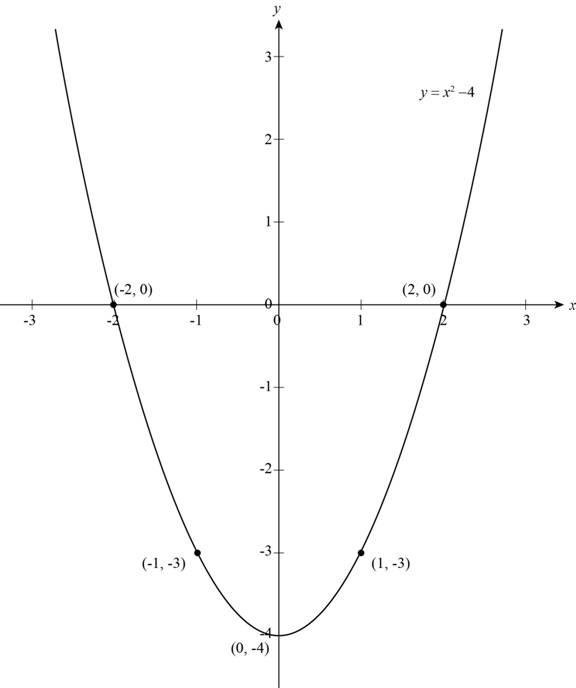# The graph of equation y = x 2 − 4 .### Precalculus: Mathematics for Calcu...

6th Edition
Stewart + 5 others
Publisher: Cengage Learning
ISBN: 9780840068071### Precalculus: Mathematics for Calcu...

6th Edition
Stewart + 5 others
Publisher: Cengage Learning
ISBN: 9780840068071

#### Solutions

Chapter 1, Problem 16T

(a)

To determine

## To sketch: The graph of equation y=x2−4 .

Expert Solution

### Explanation of Solution

The given equation is y=x24 .

Substitute different values of x to get corresponding values of y in above equation and make a table,

 x y=x2−4 (x,y) ±2 0 (2,0) , (−2,0) ±1 −3 (1,−3) , (−1,−3) 0 −4 (0,−4)

Plot points from above table and connect them by a smooth curve which is shown in Figure (1),Figure (1)

Figure (1) shows the graph of equation y=x24 that is a parabola.

(b)

To determine

### To find: The x-intercepts and y-intercepts of graph of the equation y=x2−4 .

Expert Solution

The values of x-intercepts are 2 , 2 and value of y-intercept is 4 .

### Explanation of Solution

Given:

The equation is,

y=x24 . (1)

Calculation:

Substitute 0 for y in equation (1) and solve for x to get the value of x-intercept,

0=x24x2=4x=±4x=±2

The x-intercept is 2 and 2.

Substitute 0 for x in given equation and solve for y to get the value of y-intercept,

y=(0)24y=4

The y-intercept is 4 .

The graph intersects the x-axis at x=2 , x=2 and these values of x-coordinates called as x-intercepts of graph and the graph intersect y-axis at y=4 called as y-intercept of graph shown in above Figure (1).

Thus, the values of x-intercepts are 2 , 2 and value of y-intercept is 4 .

(c)

To determine

### To check: The symmetry of graph of the equation y=x2−4 .

Expert Solution

The graph of the equation y=x24 is symmetry about y-axis.

### Explanation of Solution

The given equation is,

y=x24 . (1)

Replace y by y in equation (1) to get new equation,

y=x24

The above equation is not equivalent to equation (1) so the graph is not symmetric about the x-axis.

Replace x by x in the equation (1) to get the new equation,

y=(x)24y=x24

The above equation is equivalent to equation (1) so the graph is symmetric about y-axis shown in Figure (1),

The Figure (1) shows that the part of the graph to the left of the y-axis is the mirror image of the part to the right of the y-axis and the points (2,0) , (2,0) are reflections of each other about the y-axis.

Thus, the graph of the equation y=x24 is symmetry about y-axis.

### Have a homework question?

Subscribe to bartleby learn! Ask subject matter experts 30 homework questions each month. Plus, you’ll have access to millions of step-by-step textbook answers!